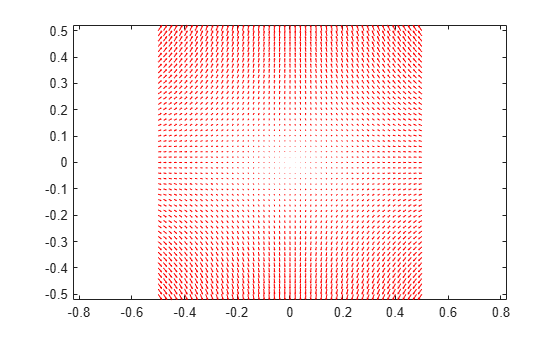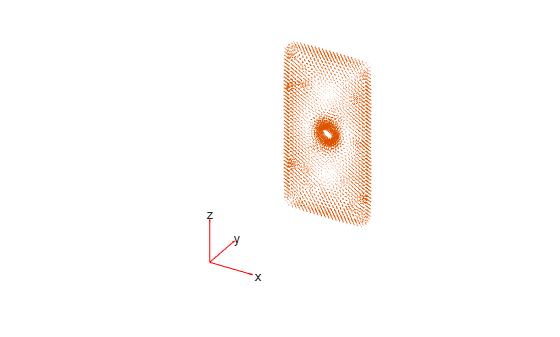# interpolateElectricFlux

Interpolate electric flux density in electrostatic result at arbitrary spatial locations

## Syntax

``Dintrp = interpolateElectricFlux(electrostaticresults,xq,yq)``
``Dintrp = interpolateElectricFlux(electrostaticresults,xq,yq,zq)``
``Dintrp = interpolateElectricFlux(electrostaticresults,querypoints)``

## Description

example

````Dintrp = interpolateElectricFlux(electrostaticresults,xq,yq)` returns the interpolated electric flux density at the 2-D points specified in `xq` and `yq`.```

example

````Dintrp = interpolateElectricFlux(electrostaticresults,xq,yq,zq)` uses 3-D points specified in `xq`, `yq`, and `zq`.```

example

````Dintrp = interpolateElectricFlux(electrostaticresults,querypoints)` returns the interpolated electric flux density at the points specified in `querypoints`.```

## Examples

collapse all

Create an electromagnetic model for electrostatic analysis.

`emagmodel = createpde("electromagnetic","electrostatic");`

Create a square geometry and include it in the model. Plot the geometry with the edge labels.

```R1 = [3,4,-1,1,1,-1,1,1,-1,-1]'; g = decsg(R1, 'R1', ('R1')'); geometryFromEdges(emagmodel,g); pdegplot(emagmodel,"EdgeLabels","on") xlim([-1.5 1.5]) axis equal```Specify the vacuum permittivity in the SI system of units.

`emagmodel.VacuumPermittivity = 8.8541878128E-12;`

Specify the relative permittivity of the material.

`electromagneticProperties(emagmodel,"RelativePermittivity",1);`

Apply the voltage boundary conditions on the edges of the square.

```electromagneticBC(emagmodel,"Voltage",0,"Edge",[1 3]); electromagneticBC(emagmodel,"Voltage",1000,"Edge",[2 4]);```

Specify the charge density for the entire geometry.

`electromagneticSource(emagmodel,"ChargeDensity",5E-9);`

Generate the mesh.

`generateMesh(emagmodel);`

Solve the model and plot the electric flux density.

```R = solve(emagmodel); pdeplot(emagmodel,"FlowData",[R.ElectricFluxDensity.Dx ... R.ElectricFluxDensity.Dy]) axis equal```Interpolate the resulting electric flux density to a grid covering the central portion of the geometry, for `x` and `y` from `-0.5` to `0.5`.

```v = linspace(-0.5,0.5,51); [X,Y] = meshgrid(v); Dintrp = interpolateElectricFlux(R,X,Y)```
```Dintrp = FEStruct with properties: Dx: [2601x1 double] Dy: [2601x1 double] ```

Reshape `Dintrp.Dx` and `Dintrp.Dy` and plot the resulting electric flux density.

```DintrpX = reshape(Dintrp.Dx,size(X)); DintrpY = reshape(Dintrp.Dy,size(Y)); figure quiver(X,Y,DintrpX,DintrpY,"Color","red")```Alternatively, you can specify the grid by using a matrix of query points.

```querypoints = [X(:),Y(:)]'; Dintrp = interpolateElectricFlux(R,querypoints);```

Create an electromagnetic model for electrostatic analysis.

`emagmodel = createpde("electromagnetic","electrostatic");`

Import and plot the geometry representing a plate with a hole.

```importGeometry(emagmodel,"PlateHoleSolid.stl"); pdegplot(emagmodel,"FaceLabels","on","FaceAlpha",0.3)```Specify the vacuum permittivity in the SI system of units.

`emagmodel.VacuumPermittivity = 8.8541878128E-12;`

Specify the relative permittivity of the material.

`electromagneticProperties(emagmodel,"RelativePermittivity",1);`

Specify the charge density for the entire geometry.

`electromagneticSource(emagmodel,"ChargeDensity",5E-9);`

Apply the voltage boundary conditions on the side faces and the face bordering the hole.

```electromagneticBC(emagmodel,"Voltage",0,"Face",3:6); electromagneticBC(emagmodel,"Voltage",1000,"Face",7);```

Generate the mesh.

`generateMesh(emagmodel);`

Solve the model.

`R = solve(emagmodel)`
```R = ElectrostaticResults with properties: ElectricPotential: [4359x1 double] ElectricField: [1x1 FEStruct] ElectricFluxDensity: [1x1 FEStruct] Mesh: [1x1 FEMesh] ```

Plot the electric flux density.

```pdeplot3D(emagmodel,"FlowData",[R.ElectricFluxDensity.Dx ... R.ElectricFluxDensity.Dy ... R.ElectricFluxDensity.Dz])```Interpolate the resulting electric flux density to a grid covering the central portion of the geometry, for `x`, `y`, and `z`.

```x = linspace(3,7,7); y = linspace(0,1,7); z = linspace(8,12,7); [X,Y,Z] = meshgrid(x,y,z); Dintrp = interpolateElectricFlux(R,X,Y,Z)```
```Dintrp = FEStruct with properties: Dx: [343x1 double] Dy: [343x1 double] Dz: [343x1 double] ```

Reshape `Dintrp.Dx`, `Dintrp.Dy`, and `Dintrp.Dz`.

```DintrpX = reshape(Dintrp.Dx,size(X)); DintrpY = reshape(Dintrp.Dy,size(Y)); DintrpZ = reshape(Dintrp.Dz,size(Z));```

Plot the resulting electric flux density.

```figure quiver3(X,Y,Z,DintrpX,DintrpY,DintrpZ,"Color","red") view([10 10])```## Input Arguments

collapse all

Solution of thermal problem, specified as an `ElectrostaticResults` object. Create `electrostaticresults` using the `solve` function.

Example: `electrostaticresults = solve(emagmodel)`

x-coordinate query points, specified as a real array. `interpolateElectricFlux` evaluates the electric flux density at the 2-D coordinate points `[xq(i) yq(i)]` or at the 3-D coordinate points `[xq(i) yq(i) zq(i)]` for every `i`. Because of this, `xq`, `yq`, and (if present) `zq` must have the same number of entries.

`interpolateElectricFlux` converts the query points to column vectors `xq(:)`, `yq(:)`, and (if present) `zq(:)`. It returns electric flux density as a column vector of the same size. To ensure that the dimensions of the returned solution are consistent with the dimensions of the original query points, use `reshape`. For example, use `DintrpX = reshape(Dintrp.Dx,size(xq))`.

Example: `xq = [0.5 0.5 0.75 0.75]`

Data Types: `double`

y-coordinate query points, specified as a real array. `interpolateElectricFlux` evaluates the electric flux density at the 2-D coordinate points `[xq(i) yq(i)]` or at the 3-D coordinate points `[xq(i) yq(i) zq(i)]` for every `i`. Because of this, `xq`, `yq`, and (if present) `zq` must have the same number of entries.

`interpolateElectricFlux` converts the query points to column vectors `xq(:)`, `yq(:)`, and (if present) `zq(:)`. It returns electric flux density as a column vector of the same size. To ensure that the dimensions of the returned solution are consistent with the dimensions of the original query points, use `reshape`. For example, use `DintrpY = reshape(Dintrp.Dy,size(yq))`.

Example: `yq = [1 2 0 0.5]`

Data Types: `double`

z-coordinate query points, specified as a real array. `interpolateElectricFlux` evaluates the electric flux density at the 3-D coordinate points `[xq(i) yq(i) zq(i)]`. Therefore, `xq`, `yq`, and `zq` must have the same number of entries.

`interpolateElectricFlux` converts the query points to column vectors `xq(:)`, `yq(:)`, and `zq(:)`. It returns electric flux density values as a column vector of the same size. To ensure that the dimensions of the returned solution are consistent with the dimensions of the original query points, use `reshape`. For example, use `DintrpZ = reshape(Dintrp.Dz,size(zq))`.

Example: `zq = [1 1 0 1.5]`

Data Types: `double`

Query points, specified as a real matrix with either two rows for 2-D geometry or three rows for 3-D geometry. `interpolateElectricFlux` evaluates the electric flux density at the coordinate points `querypoints(:,i)` for every `i`, so each column of `querypoints` contains exactly one 2-D or 3-D query point.

Example: For a 2-D geometry, ```querypoints = [0.5 0.5 0.75 0.75; 1 2 0 0.5]```

Data Types: `double`

## Output Arguments

collapse all

Electric flux density at query points, returned as an `FEStruct` object with the properties representing the spatial components of the electric flux density at the query points. For query points that are outside the geometry, `Dintrp.Dx(i)`, `Dintrp.Dy(i)`, and `Dintrp.Dz(i)` are `NaN`. Properties of an `FEStruct` object are read-only.

## Version History

Introduced in R2021a